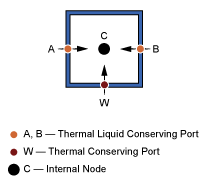## Thermal Liquid Modeling Framework

### How Blocks Represent Components

Thermal Liquid models are based on the finite volume method. This method discretizes a thermal liquid system into multiple control volumes that interact via shared interfaces. An oil pipeline system is one example: you can model this system as a set of pipeline segments that connect serially along the pipeline length.

Discretization of Pipeline SystemA control volume can represent a thermal liquid component, such as an oil pipeline, or a part of a component, such as a pipeline segment. You can discretize a thermal liquid system and its components as finely as you need, for example to increase simulation accuracy. However, the finer the discretization, the greater the model complexity—and the slower the simulation.

Thermal Liquid blocks represent the control volume of a component using an internal node. This node provides the liquid pressure and temperature inside the component. The node is not visible, but you can access its parameters and variables using Simscape™ data logging. For more information, see About Simulation Data Logging.

Simscape Nodes in Pipe (TL) BlockTwo physical principles govern the dynamic evolution of liquid pressure and temperature at the internal node of a control volume: mass conservation and energy conservation. Pressure and temperature computation is carried out for the control volume surrounding the internal node. This control volume is the total volume of the thermal liquid component the block represents.

A second set of nodes represents the interfaces through which a finite volume can interact with its neighbors. These nodes are visible as Simscape conserving ports, of which Thermal Liquid conserving ports are the most important. By allowing the exchange of mass, momentum, and energy between adjacent liquid volumes, Thermal Liquid conserving ports govern the dynamic evolution of the finite volume as it tends to a steady state.

### How Ports Represent Interfaces

Thermal Liquid conserving ports provide the liquid pressure and temperature at the interfaces they represent. They also provide the flow rates of mass and heat, which govern the interactions between thermal liquid components. Pressure and temperature are the Across variables of the Thermal Liquid domain, while the flow rates are the Through variables.

Two physical principles govern the mass and heat flow rates through a Thermal Liquid conserving port: momentum conservation and energy conservation. The mass flow rate at a port is computed from the momentum conservation principle. The heat flow rate at a port is computed from the thermal energy conservation principle.

The flow rate computations are carried out for half the control volume of a thermal liquid component. The half control volume is bounded on one end by the interface the port represents, and on another end by a parallel surface passing through the control volume centroid.

The figure shows the half control volume for flow rate computations at interface A of a pipeline segment. Interface A corresponds to Thermal Liquid conserving port A of a Pipe (TL) block. Node C corresponds to the internal node of the block, which is coincident with the control volume centroid.

Half Control Volume for Flow Rate Calculations### Full Flux Scheme

Blocks in the Thermal Liquid library implement a full flux scheme. Using this scheme, the net heat flux through a Thermal Liquid conserving port contains both convective and conductive flux contributions. By including thermal conduction in the flow direction, Thermal Liquid blocks provide more realistic simulation of the physical system they represent.

Other advantages of the full flux scheme include enhanced simulation robustness of thermal liquid models. This robustness becomes relevant in models where the conductive flux contribution can be dominant. Examples include instances of low mass flow rates and flow reversal, during which the convective flux becomes negligible or vanishes altogether.